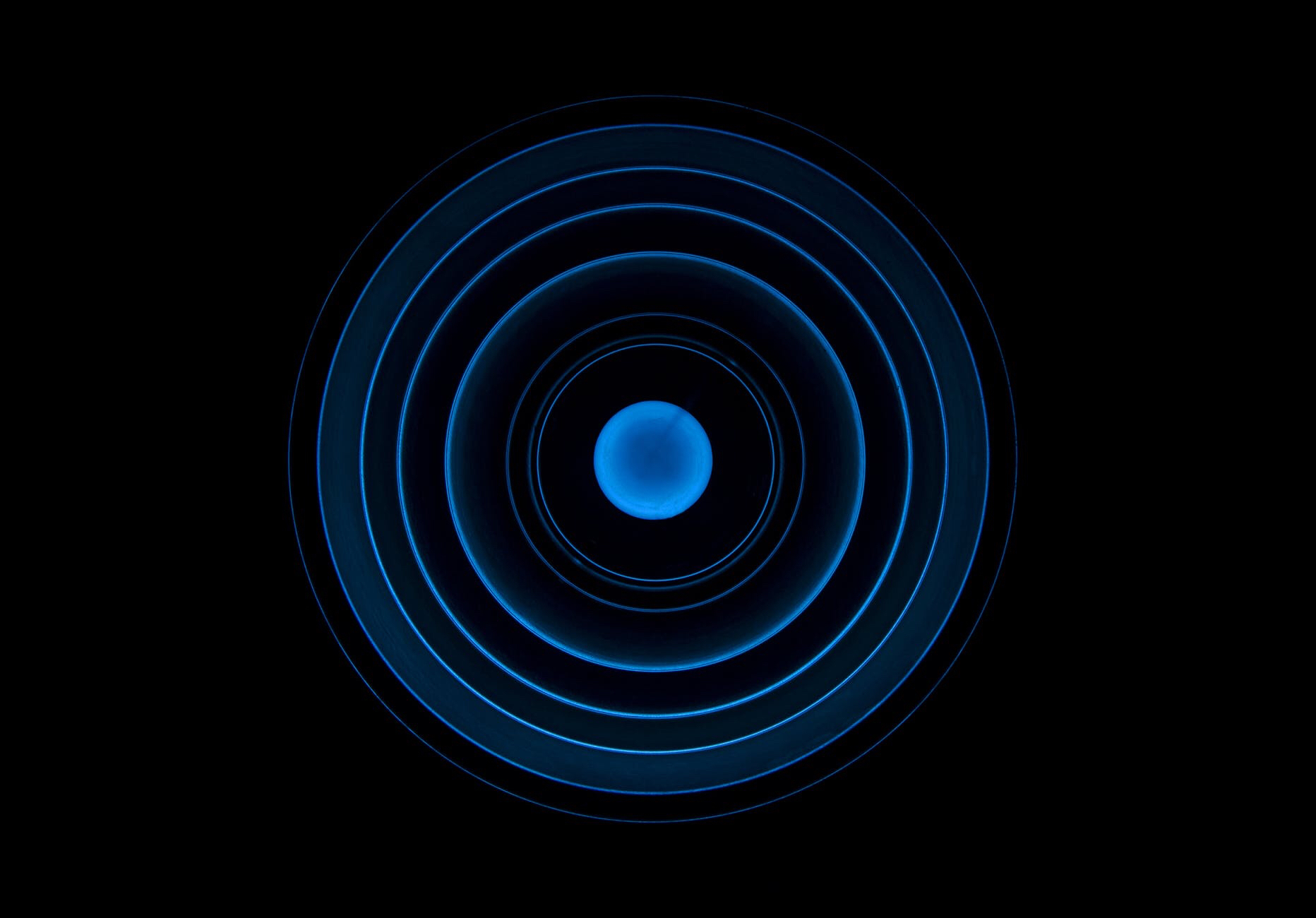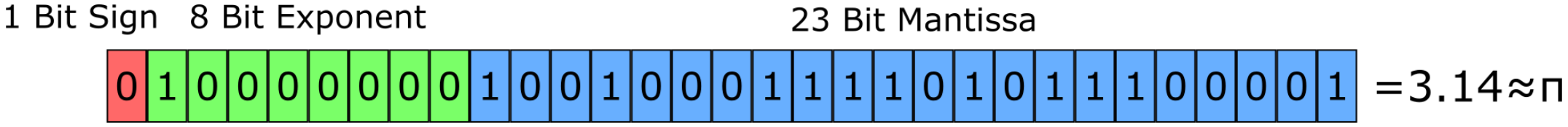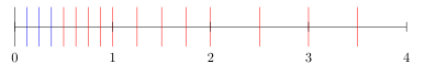# A short Introduction into IEEE 754 Floating-Point Model

Welcome back to a new post on thoughts-on-cpp.com. In today's post, I would like to talk about the floating-point model of the IEEE 754 standard. Before we start I would like to state that I'm neither a mathematician nor an expert on this topic. I'm just interested in this and I'm learning the best myself by explaining it to others. So feel free to leave comments about misunderstandings or other problems you see in the explanations of this post. As a reference I'm using the IEEE 754 standard and the book "Numerische Mathematik" which is, in my opinion, one of the best books about numerical calculation, but unfortunately only available in German.The IEEE 754 standard is defining the binary representation of floating-point numbers, this is what we focus on now, and their operations. A Floating-point number x is defined as

$$x=s \cdot m \cdot b^e$$

Where s is the sign of the number represented by one bit( which is either 1 for negative , and 0 for positive numbers), m is the mantissa (also called precision because its size defines the precision of the floating-point model) represented by p bits, b is the base of the numeral system (which is 2 for our binary numeral system on today's computers), and e is the exponent represented by r bits.

The mantissa m is defined with a precision k, the number $a_i$ (which is in a binary system 0 or 1), and $E=2$ as

$$m=a_1E^{k-1}+a_2E^{k-2}+\dots+a_{k-1}E^1+a_kE^0$$

To be able to store also negative exponents e with a positive number we need to add a bias, called B. Therefore we can say our exponent e can be stored as the positive number E with $E=e+B$. And B can be calculated by $B=2^{r-1}-1$. Typical values for single and double precision are:

TypeSizeMantissaExponentValue of ExponentBias
Single32238$-126 \leq e < 127$127
Double645211$-1022 \leq e < 1023$1023

Let's try an example now. How would be the representation of $\pi=3.14$ look like?

$$\\ 3 / 2 = 1 \rightarrow 1 \\ 1 / 2 = 0 \rightarrow 1 \\$$

$$\\ 0.14 \cdot 2 = 0.28 \rightarrow -0 \\ 0.28 \cdot 2 = 0.56 \rightarrow -0 \\ 0.56 \cdot 2 = 1.12 \rightarrow -1 \\ \cdot \\ \cdot \\ = 0.001000111101011100001 \\$$

As a result $\pi$'s binary representation is 11,00100011110101110001 and after normalization with $r-1$ and $r=8$ bit

$$\\ 11,001000111101011100001 \cdot 2^{01111111-01111111} \\ 1,1001000111101011100001 \cdot 2^{10000000-01111111} \\$$

we get the mantissa $m=1,100100011110101110001$ and an exponent with bias of $e=10000000$. Now $\pi$ looks like the following as represented in the floating point model:But wait, I remember values like 0.3 are a problem in binary representation. Is that true? Let's try.

$$\\ 0.3 \cdot 2 = 0.6 \rightarrow -0 \\ 0.6 \cdot 2 = 1.2 \rightarrow -1 \\ 0.2 \cdot 2 = 0.4 \rightarrow -0 \\ 0.4 \cdot 2 = 0.8 \rightarrow -0 \\ 0.8 \cdot 2 = 1.6 \rightarrow -1 \\ 0.6 \cdot 2 = 1.2 \rightarrow -0 \\ \cdot \\ \cdot \\ = 010010.... \\$$

Ok, that seems to be really a problem, fractions like 0.3 can't be represented exactly in a binary system because we would need an infinite number of bits and therefore rounding has to be applied while converting back from binary to a decimal representation. The machine epsilon is giving the upper bound of the rounding error and can be calculated by

$$\tau=\frac{E}{2}E^{-k}$$

What we have talked about until now is valid for normalized numbers, but what are exactly normalized numbers? And are there denormalized numbers? In short, yes there are. But let's start with normalized numbers Normalized numbers are numbers which are at least as big as the precision of the system and therefore have a leading implicit number/bit. You remember the 1,1001...? Because every normalized number has this implicit bit we can save one bit storage. Denormalized numbers on the other hand are smaller than the precision and therefore can't be represented with $x=s \cdot m \cdot b^e$ but with $x=m \cdot b^{de}$ with de as the smallest possible normal exponent. So let's have a look at how all this looks like in a number series and think about what this will tell us.By Blacklemon67 at English Wikipedia, CC BY-SA 3.0

The number series is representing all normalized numbers in red and all denormalized numbers in blue. As we can see the first red line represents the smallest (or closest) possible positive value to zero. For single precision the smallest absolute value is $2^{-126}$. For double precision the smallest absolute value is $2^{-1022}$. Also very important to point out is the fact that the distance between the numbers is not equidistant in a binary system, they are increasing by a factor of 2 with each power of two. This is one reason why comparing floating-point numbers can be a hard job.

Herewith I would like to close this post. We've had an exciting little journey into number representation of binary systems and experienced their benefits, but also drawbacks. And for sure it's not an easy topic, there is quite more to say about.

Since you've made it this far, sharing this article on your favorite social media network and giving feedback would be highly appreciated 💖!

Published